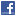## General Mathematics## Fundamental of Mathematics; Division by Zero Calculus and a New Axiom

Authors: Saburou Saitoh

Based on the preprint survey paper (\cite{sur}), we will discuss the theoritical point of the division by zero calculus. We will need a new axiom for our mathematics. The contents in this paper seem to be serious for our mathematics and for our world history with the materials in \cite{sur}. So, the author hopes that the related mathematicians, mathematical scientists and others check and consider the topics from various viewpoints.

Comments: 18 Pages. The contents in this paper seem to be serious for our mathematics and for our world history with the materials in \cite{sur}. So, the author hopes that the related mathematicians, mathematical scientists and others check and consider the topics from vari